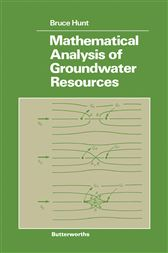# Mathematical Analysis of Groundwater ResourcesUS\$72.95
Mathematical Analysis of Groundwater Resources focuses on groundwater flow.
The book first discusses the scope of the study, definition of terms, and mathematical preliminaries. The text examines the equations of groundwater flow. Continuum concepts; flux and pore velocities; Darcy's Law for Anisotropic Aquifers; Conservation of Mass equations; and boundary conditions are discussed. The book also underscores the formulation of boundary-value problems. Regional problems, confined flow problems, sea water intrusion problems, and free surface flows are discussed.
The text also looks at the approximate solution of boundary-value problems, inverse problems, and groundwater pollution. The book then presents the exact solutions of steady-flow problems. Problem formulations; analytic coordinate transformations; analytic functions of a complex variable; applications of the Schwarz-Christoffel transformation; and superposition of solutions are described. The text also discusses the exact solution of unsteady problems. The Laplace transform, groundwater recharge problems, well storage effect, and two well recovery problems are discussed.
The book is a good source of data for researchers who are interested in groundwater flow.
• Elsevier Science; January 2016
• ISBN: 9781483103075
• Read online, or download in secure PDF format
• Title: Mathematical Analysis of Groundwater Resources
• Author: Bruce Hunt
• Imprint: Butterworth-Heinemann